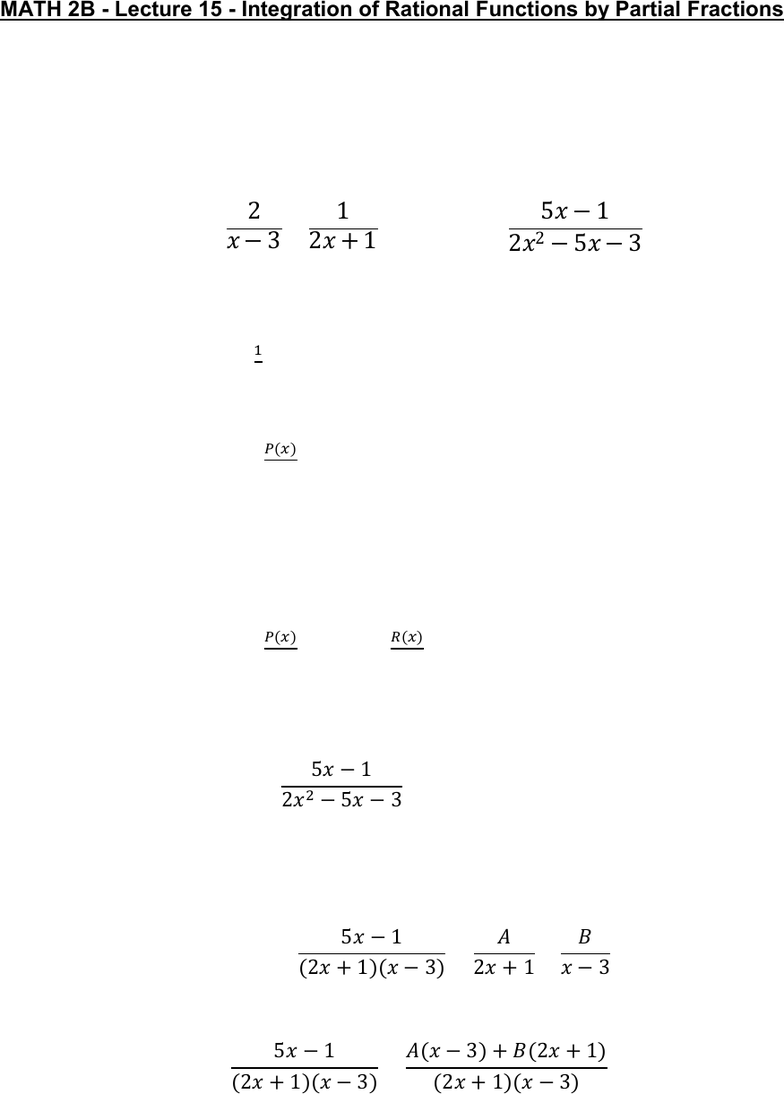Class Notes (1,100,000)
US (490,000)
UC-Irvine (20,000)
MATH (1,000)
MATH 2B (600)
Lecture 15

# MATH 2B Lecture 15: Integration of Rational Functions by Partial FractionsPremium

Department
Mathematics
Course Code
MATH 2B
Professor
ERJAEE, G.
Lecture
15

This preview shows page 1. to view the full 4 pages of the document.02/08/2019
MATH 2B - Lecture 15 - Integration of Rational Functions by Partial Fractions
We learn how to integrate any rational function (ratio of polynomials) by expressing it as
a sum of several simpler-functions that we already know how to integrate. These
simpler functions are called partial fractions.
Example:


Solution:
Solving the left-hand side, we obtain the answer:


Solving the right-hand side directly is not easy:
In general, we consider a rational function

 P(x), Q(x) are polynomials
We can only break f(x) into sum of partial fractions when 
(deg: degree of polynomials)
If , then f(x) is improper, we then need the long division
to divide Q into P until a remainder R(x) is obtained such that 


 
 (long division)
Now, let’s try to solve the integral on the right-hand side by using partial
fractions
1. Factor denominator Q(x)



2. Sum of partial fractions
We assume f(x) can be expressed as:

3. Compute unknown A, B by adding partial fractions